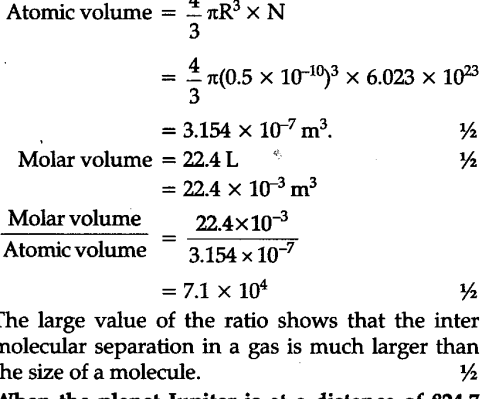# One mole of an ideal gas at NTP and pressure occupies 22.4 L (molar volume)

One mole of an ideal gas at NTP and pressure occupies 22.4 L (molar volume). What is the ratio of molar volume to the atomic volume of a mole of hydrogen ? (Take the size of hydrogen molecule to be about 1 \$A\overset{0}{\mathop{{}}},\$). Why is this ratio so large ?